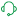# App Development

Discussion board where members can learn more about Qlik Sense App Development and Usage.

Announcements
April 22, 2PM EST: Learn about GeoOperations in Qlik Sense SaaS READ MORE
cancel
Showing results for
Search instead for
Did you mean:Partner

## Filter on Calculated Variable in Set Analysis

Hi All,

I'm constructing a table for our travel team, analyzing the expense cities to where we travel.
One of the calculations i make is suggestion new expensive cities to be considered in our travel policy. (the result of the measure is a 1 or a 0, the variable is called vNewExpensive

Now i would like to filter on these in set analysis for my further calculations.
Currently I have something like this: [DestinationCity] = {"=\$(=\$(vNewExpensive))<1"} in my set analysis, but it doesn't seem to work

vNewExpensive has the following expression:
if(
//Service Length has to be longer than 20
Sum({< [ReferenceDate.Calendar.DateNum] = {">=\$(=\$(SelectedDateMinYTM))<\$(=\$(SelectedDateMaxYTM))"},ExpenseFlag={'0'}>} ServiceLength) >= 20
AND
//# Booking shas to be bigger than 0
Count({< [ReferenceDate.Calendar.DateNum] = {">=\$(=\$(SelectedDateMinYTM)) <\$(=\$(SelectedDateMaxYTM))"},ExpenseFlag={'0'}>} Distinct ReservationNumber) >= 0
AND
//Difference between the Q3 of this destination and the 'Overall Q3' has to be bigger than 0
//Normal 3rd Quartile
Avg(Aggr( NoDistinct
Fractile( {< [ReferenceDate.Calendar.DateNum] = {">=\$(=\$(SelectedDateMinYTM)) <\$(=\$(SelectedDateMaxYTM))"},ExpenseFlag={'0'}>} ClientNormalizedPrice,0.75)
,[DestinationCity]))
-
//Overall 3rd Quartile
Avg(Aggr( NoDistinct
Fractile( {< [ReferenceDate.Calendar.DateNum] = {">=\$(=\$(SelectedDateMinYTM)) <\$(=\$(SelectedDateMaxYTM))"},ExpenseFlag={'0'}>} ClientNormalizedPrice,0.75)
,"Service SubCategory")) > 0
,1,0)

Hoping anybody can help
Greetings Yorick

3 RepliesMVP

@Yorick_Stevens  I don't think set analysis will work here but you can use expression like below

if(
//Service Length has to be longer than 20
Sum({< [ReferenceDate.Calendar.DateNum] = {">=\$(=\$(SelectedDateMinYTM))<\$(=\$(SelectedDateMaxYTM))"},ExpenseFlag={'0'}>} ServiceLength) >= 20
AND
//# Booking shas to be bigger than 0
Count({< [ReferenceDate.Calendar.DateNum] = {">=\$(=\$(SelectedDateMinYTM)) <\$(=\$(SelectedDateMaxYTM))"},ExpenseFlag={'0'}>} Distinct ReservationNumber) >= 0
AND
//Difference between the Q3 of this destination and the 'Overall Q3' has to be bigger than 0
//Normal 3rd Quartile
Avg(Aggr( NoDistinct
Fractile( {< [ReferenceDate.Calendar.DateNum] = {">=\$(=\$(SelectedDateMinYTM)) <\$(=\$(SelectedDateMaxYTM))"},ExpenseFlag={'0'}>} ClientNormalizedPrice,0.75)
,[DestinationCity]))
-
//Overall 3rd Quartile
Avg(Aggr( NoDistinct
Fractile( {< [ReferenceDate.Calendar.DateNum] = {">=\$(=\$(SelectedDateMinYTM)) <\$(=\$(SelectedDateMaxYTM))"},ExpenseFlag={'0'}>} ClientNormalizedPrice,0.75)
,"Service SubCategory")) > 0,

[DestinationCity]  )Partner
Author

Hi Kush,

How would you u suggest to filter on the following measure

Avg(Aggr( NoDistinct
Fractile( {< [ReferenceDate.Calendar.DateNum] = {">=\$(=\$(SelectedDateMinYTM)) <\$(=\$(SelectedDateMaxYTM))"},ExpenseFlag={'0'}>} ClientNormalizedPrice,0.75)
,"Service SubCategory"))

Thanks in advanceSupport (Former)

@Kush Any chance you can swing back by on this one to check the last update by the poster to see if you can add any further guidance?  Thanks in advance as always and sorry we are still having issues with notifications.

Regards,
Brett

To help users find verified answers, please do not forget to use the "Accept as Solution" button on any post(s) that helped you resolve your problem or question.
I now work a compressed schedule, Tuesday, Wednesday and Thursday, so those will be the days I will reply to any follow-up posts.